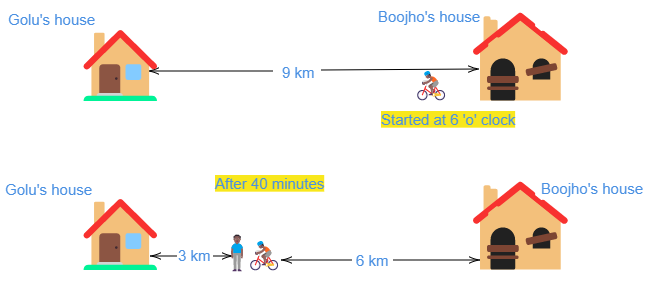# Distance between Bholu’s and Golu’s house is 9 km. Bholu has to attend Golu’s birthday party at 7 o’clock. He started from his home at 6 o’clock on his bicycle and covered a distance of 6 km in 40 minutes. At that point he met Chintu and he spoke to him for 5 minutes and reached Golu’s birthday party at 7 o’clock. With what speed did he cover the second part of the journey? Calculate his average speed for the entire journey.

Start time $=6\ 'o'\ clock$

Party time $7\ 'o'\ clock$

Distance between Golu's house and Boojho's house $=9\ km$

Distance covered in 40 minutes $=6\ km$

Time taken to cover first journey $=$40\ minute

Time spent talking Chintu $=$5 minute

Total time taken $=40+5$=45 minute

Time left $=$1 hour$-$45 minutes

$=$15 minute=\frac{15}{60}\ hour

Distance remained to cover $=9\ km-6\ km=3\ km$

Therefore, speed at second journey $=\frac{distancce}{time}$

$=\frac{3\ km}{\frac{15}{60}}$

$=12\ km/h$